# Particle decay, Lorentz transformations with angles

• Cocoleia
I'm missing somethingFor the momentum components of the daughter particle in the second situation, I am a bit lost. Would I have to be using something like this and then I guess the momentum would only vary on one axis? I am confused.It seems you are saying that the mother particle is at rest in the first frame. If that is the case, you can determine the velocity of the daughter particle is the first frame. That gives the velocity of the daughter particle in the second frame.It seems you are saying that the mother particle is at rest in the first frame. If that is the case, you can determine the velocity of the daughter particle is the first frame. That gives the velocity of the daughter particlef

## Homework Statement

I have a mother particle at rest, which decays to a daughter particle. The daughter has mass m, momentum p and energy E and is at an angle θ1.

Now I have to assume that the daughter is emitted at an angle θ2, and the mother is moving along the x-axis with velocity βc. I need to find a relation between the two angles, as well as the momentum components of the daughter particle in the second situation.

## Homework Equations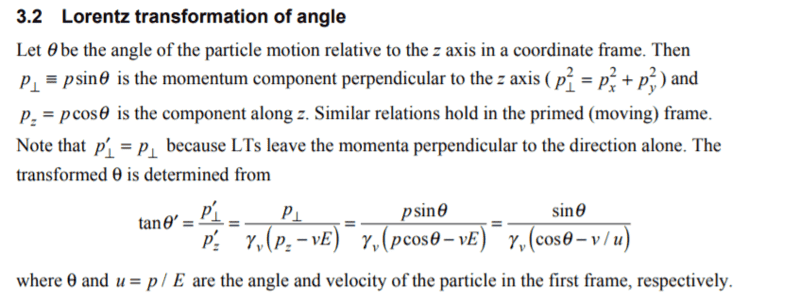## The Attempt at a Solution

I think I could use this relation to find the relation between the angles. However, I don't know the velocity of the daughter particle in the second situation. I'm missing something

For the momentum components of the daughter particle in the second situation, I am a bit lost. Would I have to be using something like this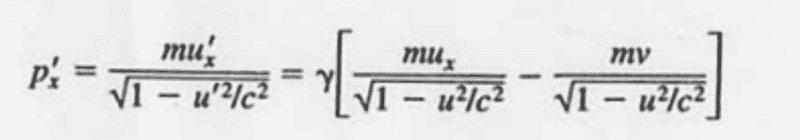and then I guess the momentum would only vary on one axis? I am confused.

#### Attachments

It seems you are saying that the mother particle is at rest in the first frame. If that is the case, you can determine the velocity of the daughter particle is the first frame. That gives the velocity of the daughter particle in the second frame.

It seems you are saying that the mother particle is at rest in the first frame. If that is the case, you can determine the velocity of the daughter particle is the first frame. That gives the velocity of the daughter particle in the second frame.
Okay, so then I would have that and my angle relation would be set.

For the momentum components, I have been reading about Four-Vectors. Is this pertinent in this situation ?

The relevance of four-vectors depends on what the problem is that you are trying to solve (you haven't said). If you need the momentum in the second frame then four-vectors would be relevant. If you just need the velocity in the second frame then I don't think you need four-vectors. What is the problem statement? Also, what did you conclude for the velocity of the daughter particle in the first frame?

The relevance of four-vectors depends on what the problem is that you are trying to solve (you haven't said). If you need the momentum in the second frame then four-vectors would be relevant. If you just need the velocity in the second frame then I don't think you need four-vectors. What is the problem statement?
I need to find a relation between the angle in the first frame and the angle in the second frame. I also need the components of the momentum in the second frame (of the daughter)

Also, what did you conclude for the velocity of the daughter particle in the first frame?

I figure it would simply be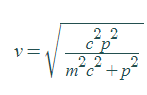If I solve for v from the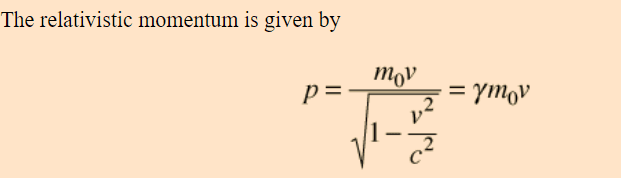(I haven't learned this in school and now I'm working on a project which requires these calculations, sorry if I'm a bit slow at it)

#### Attachments

You should apply conservation of momentum in the first frame. The momentum of the daughter particle is equal to the momentum of the mother particle, if there are no other particles involved.

You should apply conservation of momentum in the first frame. The momentum of the daughter particle is equal to the momentum of the mother particle, if there are no other particles involved.
there are no others.

Isn't the momentum of the mother just 0 because it is at rest ?

Yes

Yes

So the velocity is fine, then conservation of momentum for the second part of my question.

What is it that you are trying to compute or determine, please?

What is it that you are trying to compute or determine, please?
I need to find the momentum of the daughter particle in the second frame, when the mother is moving, and express it in terms of the momentum/energy/angle of the first frame

If the velocity of the daughter particle is zero in the first frame, you can immediately get its velocity in the second frame. You don't really need a formula, but if you want to use a formula, there is the velocity addition formula.

Or you can use four-vectors, writing the four-vector of the daughter particle in the first frame and applying the Lorentz transformation to it. But I think it is simpler to just write the velocity in the second frame and then get its momentum from the velocity using the relativistic momentum formula.

I have a mother particle at rest, which decays to a daughter particle. The daughter has mass m, momentum p and energy E and is at an angle θ1.
Note that this implies there are other particles besides the daughter particle, otherwise momentum wouldn't be conserved.

I think I could use this relation to find the relation between the angles. However, I don't know the velocity of the daughter particle in the second situation. I'm missing something.
It's generally a good idea to avoid working with velocities and stick with energy and momentum. Because energy and momentum are the components of the momentum four-vector, you can use the Lorentz transformations to calculate their values in a different frame.

I guess the momentum would only vary on one axis? I am confused.
Right. The y and z components of the momentum are the same in the two frames because they're perpendicular to the direction of the boost. It's just like how length contraction only happens along the direction of motion.

It's generally a good idea to avoid working with velocities and stick with energy and momentum. Because energy and momentum are the components of the momentum four-vector, you can use the Lorentz transformations to calculate their values in a different frame.
So, am I basically just using this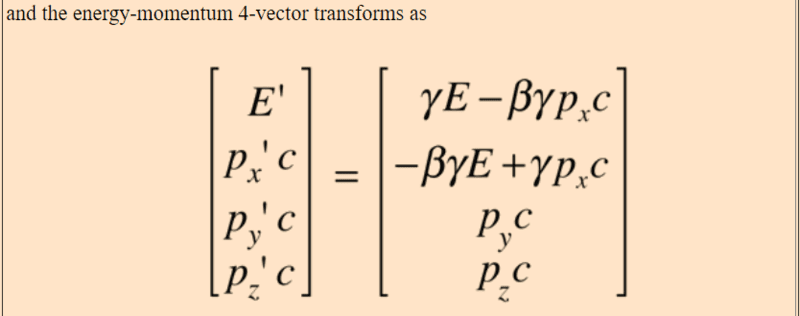Where I can find the components px, py and pz from the first frame using geometry (psin and pcos) and then just have to solve for beta and gamma ?

#### Attachments

Note that this implies there are other particles besides the daughter particle, otherwise momentum wouldn't be conserved.

So what is the story on this reaction? If you have angles there must be more than one daughter particle.

So what is the story on this reaction? If you have angles there must be more than one daughter particle.
My supervisor never specified he just gave this as an example and never mentioned more than one particle. It was just for kinematics there was no specific decay defined (I had in the past lambda decaying to pion and proton)

Well, either there are more than one daughter particle or all the angles are zero.

Well, either there are more than one daughter particle or all the angles are zero.
Just to clarify, in the second frame
The mother particle is moving, and then decays. So wouldn't the momentum just be the exact same if there was no other particle?

I don't understand your question, my bad. If the daughter particle moves off at an angle then there has to be a second daughter particle present to cancel out its momentum perpendicular to the mother particle. (The total momentum perpendicular to the motion of the mother particle is zero.)

So what is the story on this reaction? If you have angles there must be more than one daughter particle.

Well, either there are more than one daughter particle or all the angles are zero.
The angle wouldn't be zero. It would be undefined. If the daughter particle didn't move off in some direction, there's no angle to have a measure for. An angle of 0, on the other hand, would mean the daughter particle moved off in the +x direction (in the original particle's rest frame).

Well yes, @vela is correct. If there is only one daughter particle then there is no angle in the first frame of reference. In the second frame of reference, the momentum would correspond to the relative velocity between the two frames of reference.